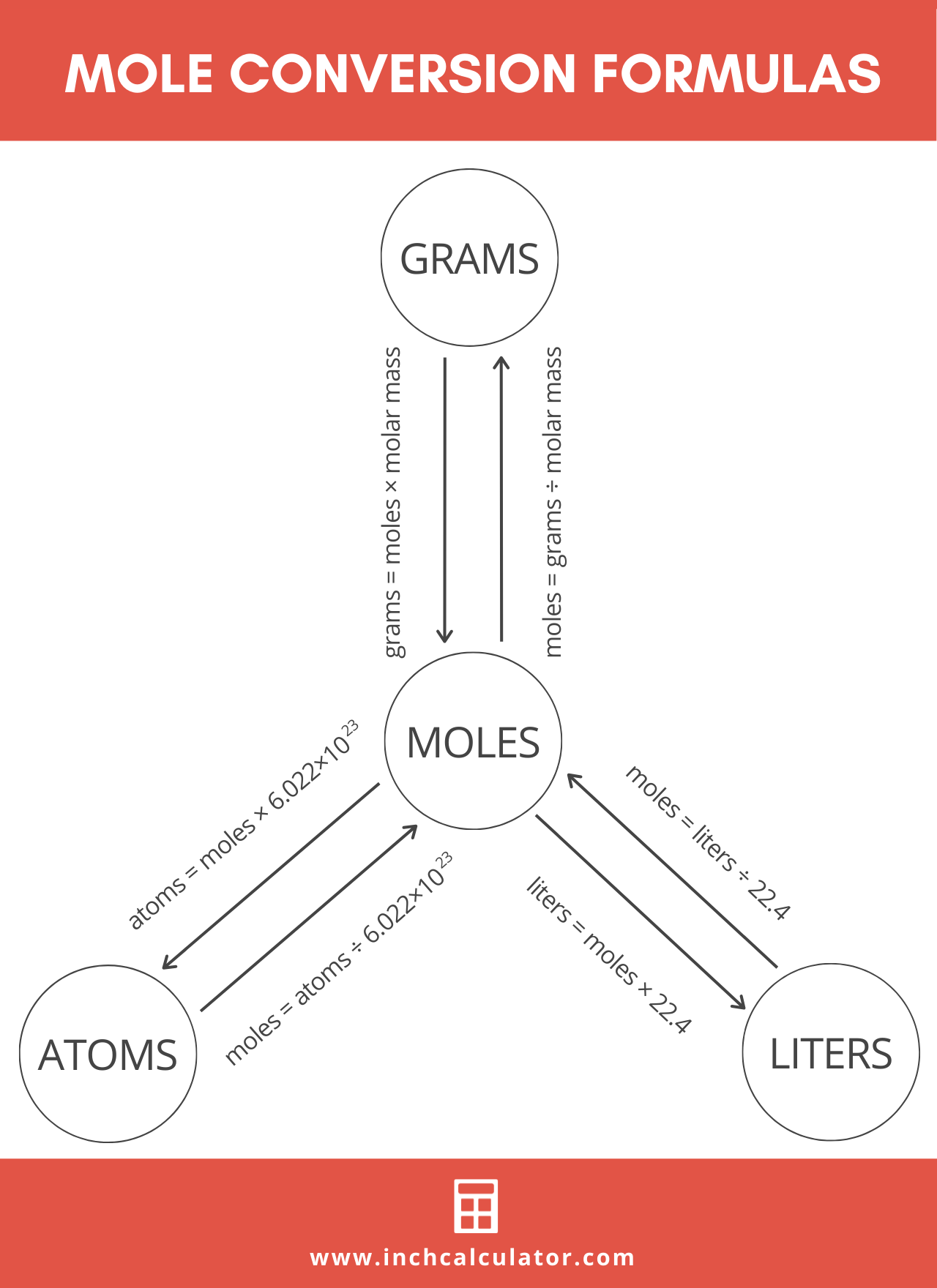# Mole Calculator

Use the mole calculator below to find the quantity of a substance using a chemical formula or known molar mass.

grams
g/mol
grams

## Quantity:

moles

### Molar Mass

g/mol
Learn how we calculated this below

## What is a Mole?

The mole, abbreviated mol, is the SI base unit to measure the amount or quantity of a substance.

It’s used as a standard measurement for a very large quantity of very small units of matter. One mole is equal to 6.02214076 × 1023 elementary entities, such as atoms, molecules, electrons, or ions.

This numerical value is known as the Avogadro constant or Avogadro’s number. Because it’s such a large number, scientific notation is almost always used to represent it.

## How to Calculate Moles

Since the mole is a measure of quantity, all substances have the same number of atoms, molecules, and other particles in one mole of the substance.

You might be wondering how you can calculate the number of moles given a mass, volume, or amount of substance. Follow along, and we’ll show you how to convert mass to moles in just a few steps. Below we’ll also cover how to convert volume and molecules to moles as well.

This conversion is useful because most chemicals are stored and measured either by weight (g) or volume (L). However, most chemical equations are written in terms of moles so that the number of atoms of each type balances out on both sides of the equation.

Using moles in chemical equations shows exactly how the reactants transform to make the products. Therefore, you need to be able to convert from weight and volume to moles and vice versa to conduct experiments in a laboratory or carry out reactions to manufacture products in industry.### Step One: Calculate Molar Mass

The first step to finding the amount of moles of substance is to determine the molar mass of the chemical formula. Molar mass is often expressed as g/mol.

You can calculate molar mass by identifying the atoms in the molecular formula, then adding the atomic mass of each element together to find the molecular weight. The atomic mass can be found on the periodic table.

For example, let’s find the molar mass of water (H2O).

H2O is composed of two hydrogen (H) atoms and one oxygen (O) atom.

The atomic mass of hydrogen is 1.00794 and that of oxygen is 15.9994. Since there are two hydrogen atoms per molecule and one oxygen atom, the formula should look like this:

molar mass (g) = 1.00794 g + 1.00794 g + 15.9994 g

Therefore, the molar mass of H2O is 18.01528 g.

Of course, if you don’t have the molar mass handy and want to skip this step, you can use our molar mass calculator to easily calculate the molecular weight of a substance.

### Step Two: Convert Mass to Moles

Now that you’re armed with the molar mass of the substance, you can calculate the quantity in moles using the total mass in grams.

#### Mass to Moles Formula

Calculate the moles n of the substance using the following formula:

n = m / M

The quantity of substance n in moles is equal to the mass m in grams divided by the molar mass M in g/mol.

For example, let’s calculate the quantity in moles equal to 120 grams of aluminum oxide, Al2O3. Note that the molar mass of aluminum oxide is 101.96 g/mol.

n = 120 g / 101.96
n = 1.18 mol

So, in this example, the quantity of 120 grams of aluminum oxide equals 1.18 moles.

You can use the calculator above to calculate this more easily or even our grams to moles calculator to quickly convert grams to moles and moles back to mass.

## How to Convert Gas Volume to Moles

The process for converting the volume of an ideal gas to moles is a little different than for mass. You’ll still use a formula, but you do not need the molar mass of the gas for the conversion.

mol = volume (L) / 22.4

The quantity of a substance in moles is equal to the volume in liters of the ideal gas divided by 22.4 L/mol.

This relationship between liters and moles holds true at standard temperature and pressure (STP) since both temperature and pressure affect the volume of a gas. This convention is used for most calculations involving gasses and is intended to represent conditions at sea level.

You can also use our liters to moles calculator for this conversion.

## How to Convert Molecules to Moles

The formula for converting molecules to moles is a little different than mass and volume because both molecules and moles are measures of quantity:

mol = molecules / 6.02214076 × 1023

The quantity of substance in moles is equal to the number of molecules divided by the Avogadro constant (6.02214076 × 1023).

Our atoms to moles calculator easily converts atoms or molecules to moles.

## References

1. International Bureau of Weights and Measures, SI base unit: mole (mol), https://www.bipm.org/en/si-base-units/mole